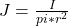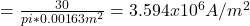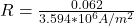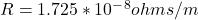## To learn to apply the concept of current density and microscopic Ohm’s law. A “gauge 8” jumper cable has a diameter d of 0.326 cm. The cable

Question

To learn to apply the concept of current density and microscopic Ohm’s law. A “gauge 8” jumper cable has a diameter d of 0.326 cm. The cable carries a current I of 30.0 A. The electric field E in the cable is 0.062 V/m. Below is a list of some common metals with their:____________

in progress 0
6 months 2021-08-03T01:28:27+00:00 2 Answers 101 views 0

1. Complete question:

To learn to apply the concept of current density and microscopic Ohm’s law.

A “gauge 8” jumper cable has a diameter of 0.326 centimeters. The cable carries a current of 30.0 amperes. The electric field in the cable is 0.062 newtons per coulomb.

What is the material of the cable?

A. Copper

B. Silver

C. Aluminum

D. Gold

E. Steel

Explanation:

We are given:

•diameter d= 0.0326cm = 0.00326m

• Current I = 30A

• Electric field e = 0.062v/m

To find the material of cable, we’ll need to find the resistivity of the material using the formula:

R = e/J

We already know e = 0.062v/m

To find current density J, we have:But r = d/2 = 0.00326 / 2 = 0.00163Hence resistivity will be,

R = e/J =From the resistivity of the material we can conclude that it is made of Copper

Resistivity = (1.726 × 10⁻⁸) Ωm

Comparing this answer with the resistivity of the listed materials in the question, this resistivity matches that of Copper the most.

Hence, the material is Copper.

Option A is correct. Copper.

Explanation:

Given,

I = 30.0 A

d = 0.326 cm = 0.00326 m

E = 0.062 V/m

The current density for the wire is given as

J = (I/A)

where J = current density = ?

I = current = 30.0 A

A = Cross sectional Area of the wire = (πd²/4) = [π×(0.00326²)/4] = 0.0000083503 m²

J = (30 ÷ 0.0000083503) = 3,592,685.3 A/m²

On a microscopic scale, Ohm’s law can be stated as

E = Jρ

where E = Electric field = 0.062 V

J = Current density = 3,592,685.3 A/m²

ρ = Resistivity of the material = ?

ρ = (E/J)

ρ = 0.062 ÷ 3,592,685.3 = (1.726 × 10⁻⁸) Ωm

Comparing this answer with the resistivity of the listed materials in the question, this resistivity matches that of Copper the most.

Hence, the material is Copper.

Hope this Helps!!!